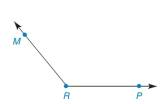Chapter 1.4, Problem 35E### Elementary Geometry for College St...

6th Edition
Daniel C. Alexander + 1 other
ISBN: 9781285195698

#### Solutions

Chapter
Section### Elementary Geometry for College St...

6th Edition
Daniel C. Alexander + 1 other
ISBN: 9781285195698
Textbook Problem
1 views

# In Exercises 33 to 40, use only a compass and a straightedge to perform the indicated constructions.Exercises 3 3 - 3 5 Given: Obtuse ∠ M R P Construct: Rays R S → , R T → , and R U → so that ∠ M R P isDivided into four ≅ angles.

To determine

To construct:

The three rays that divide MRP into four congruent angles.

Explanation

Given:

The obtuse MRP is given.

The given figure is,

Figure (1)

Approach:

Use compass to make an arc that intersect both sides of MRP at point G and H as shown in figure (2).

Figure (2)

With G and H as centre mark two arcs equal radii to intersect at an interior point J of MRP.

Draw a ray from RT shown in figure (3)

### Still sussing out bartleby?

Check out a sample textbook solution.

See a sample solution

#### The Solution to Your Study Problems

Bartleby provides explanations to thousands of textbook problems written by our experts, many with advanced degrees!

Get Started

#### Find limx1x2+4x+3x2+3x+2

Applied Calculus for the Managerial, Life, and Social Sciences: A Brief Approach

#### I=ER,E=120,andR=12.findI.

Elementary Technical Mathematics

#### True or False: div curl F = 0.

Study Guide for Stewart's Multivariable Calculus, 8th

#### True or False: is a convergent series.

Study Guide for Stewart's Single Variable Calculus: Early Transcendentals, 8th

#### Simplify. (16+xyx)+(x)

Mathematics For Machine Technology